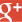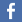﻿ Convert text to hexadecimal, base64 or binary online ﻿

# Base 64 / Binary / Text / Hexadecimal Online Converter## Base 64 / Hexadecimal / decimal / binary online Converter :

### Binary conversion :

When we learn to count, we obvisouly learn base 10 (decimal system), but computers always count in base 2 (binary system). Computer transistors can only handle two states, activate or desactivate, which corresponds to an electrical current. The 1 stands for an activate state, which is a non-null electrical current, while the 0 stands for a null current.
To convert a text to a binary representation, we first have to convert all the letters into their decimal representation. What we see on the keyboard isn't what the computer sees. Each key corresponds to a specific number in decimal base. For instance, the letter "a" is represented by the number 97 on your computer. If we take the word "hello", we would first have to convert it into decimal representation, which would give according to ASCII tables :
104, 101, 108, 108, 111
Once we did this conversion, we're going to convert each number in binary representation. For that, we take the number and apply to it a division by 2. For each division we take the rest (thanks to modulo operation), and that rest will be part of the binary representation. Then we did another division by 2, and so on until we reach 0. Division rests will be the binary representation, which we'll have to invert because we want to start from 0. For instance for "a" letter, which is 97 :

97 % 2 = 1
48 % 2 = 0
24 % 2 = 0
12 % 2 = 0
6  % 2 = 0
3  % 2 = 1
1  % 2 = 1

Here we can see that the binary representation for "a" is 1100001 (we reverse the code). You can see that "97" binary representation is only 7 bits long. That's because we don't need the last one, because 97 isn't big enough to use it. To check if that's true, you have to know that each bit in a byte belongs to a specific decimal value. Beginning at the left side of the binary code, first bit is 128, then 64, 32, 16, 8, 4, 2 and 1. in our representation of "a" letter , we can see that bits 64, 32 and 1 are activate. 64 + 32 + 1 = 97. On this webpage, that's the way we convert text to binary. To convert Hexadecimal to Binary, we first convert the Hexadecimal to Decimal, then we use the same function as explained before to convert the decimal values to binary data.

Hexadecimal representation (litteraly base 16) is a very handfull notation for computers because it's more compact than decimal to store numbers (for instance 2 000 000 in decimal will be 1E8480 in hexadecimal). Moreover, hexadecimal digits exactly corresponds to 4 bits, which allow to represent a byte with 2 hexadecimal digits. This will ease the calculations.
Hexadecimal to binary conversion is actually very simple. You just have to split the byte in 2 4-bits parts, then assign to each 4-bits group its hexadecimal value (as I said, hexadecimal digits corresponds to 4 bits). For instance for our letter "a" :

Binary :       1100 | 0001

Here we take "0110" which equal to 6 in decimal, 6 in hexadecimal, and "0001" which corresponds to 1 in both decimal and hexadecimal. That gives us : "61".

### Base 64 conversion :

Base64, as says its name, is a base 64 way of seeing the data. Instead of counting from 1 to 10, or 1 to 16, we'll have a 64 caracters alphabet, plus a 65th which is the "=" (equal) sign. This last caracter is only used to terminate a base64 sequence. The base 64 alphabet goes from 0 (A in base 64), to 63 (/ in base 64). A base 64 sequence will be composed of caracters : A-Z, a-z, 0-9, + and /. Base 64 uses a 6 bits representation, because you can represent up to 64 different things with 6 bits Bytes.

When you convert a text to Base 64 using our tool, it will be first separate into chunks of 24 bits, which is 3 Bytes. Then it will encode each 6 bits of the input with its base 64 value. For instance, let's say we want to encode the word "md5" :

ASCII         |       m       |        d        |         5       |
Binary     |011011 01|0110 0100|00 110101|
Base 64    |     b     |     W      |    Q      |      1     |

If the input's amount of bits isn't a multiple of 6, base 64 complete it with 0 bits. For instance if you submit "ABCD", we'll try to separate it by chunks of 24 bits, what remains is a Byte, so 8 bits. We will take 6 bits out, and will remain 2 bits. Now we just have to complete those 2 bits to reach another 6 bits. We'll add 4 "0" bits to obtain a final multiple of 6, then we add "=" signs to reach the 24 bits multiple.

You can know how much bytes contained the last group of a base 64 sequence by looking to the number of "=" at the end of it. For instance with our last example, we'll have to add two "=" to obtain four 6 bits caracters (the first one was the first 6 bits of letter "D", the second one the last 2 bits of letter "D" plus the four "0" bits we added). If we had two remaining bytes in our last chunk, we would only need one "=" sign to complete the sequence. That's how you convert text to Base 64.

You will also find an option to convert base 64 to hexadecimal values, that's usefull for some hashes that were directly converted to base64 instead of usual ascii representation. This option was made to convert your base 64 to sha 1, 256, 512, or any kind of hash. Then you'll just have to type those hashes on our database.

﻿Mentions légales | 24,481,155 cracked hashes since 11/2015 | There are words in the database |